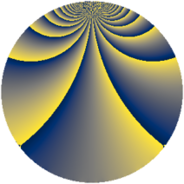Properties

 Label 3969.1.caLevel $3969$ Weight $1$ Character orbit 3969.ca Rep. character $\chi_{3969}(514,\cdot)$ Character field $\Q(\zeta_{42})$ Dimension $12$ Newform subspaces $1$ Sturm bound $504$ Trace bound $0$

Related objects

Defining parameters

 Level: $$N$$ $$=$$ $$3969 = 3^{4} \cdot 7^{2}$$ Weight: $$k$$ $$=$$ $$1$$ Character orbit: $$[\chi]$$ $$=$$ 3969.ca (of order $$42$$ and degree $$12$$) Character conductor: $$\operatorname{cond}(\chi)$$ $$=$$ $$441$$ Character field: $$\Q(\zeta_{42})$$ Newform subspaces: $$1$$ Sturm bound: $$504$$ Trace bound: $$0$$

Dimensions

The following table gives the dimensions of various subspaces of $$M_{1}(3969, [\chi])$$.

Total New Old
Modular forms 156 36 120
Cusp forms 12 12 0
Eisenstein series 144 24 120

The following table gives the dimensions of subspaces with specified projective image type.

$$D_n$$ $$A_4$$ $$S_4$$ $$A_5$$
Dimension 12 0 0 0

Trace form

 $$12 q - q^{4} - q^{7} + O(q^{10})$$ $$12 q - q^{4} - q^{7} + 3 q^{13} + q^{16} - 2 q^{25} + q^{28} - 3 q^{31} - 13 q^{37} + q^{43} + q^{49} + 7 q^{52} - 11 q^{61} + 2 q^{64} - q^{67} - q^{79} + 3 q^{97} + O(q^{100})$$

Decomposition of $$S_{1}^{\mathrm{new}}(3969, [\chi])$$ into newform subspaces

Label Dim. $$A$$ Field Image CM RM Traces $q$-expansion
$a_{2}$ $a_{3}$ $a_{5}$ $a_{7}$
3969.1.ca.a $12$ $1.981$ $$\Q(\zeta_{21})$$ $D_{42}$ $$\Q(\sqrt{-3})$$ None $$0$$ $$0$$ $$0$$ $$-1$$ $$q-\zeta_{42}^{10}q^{4}+\zeta_{42}^{19}q^{7}+(\zeta_{42}^{4}-\zeta_{42}^{6}+\cdots)q^{13}+\cdots$$